﻿ 基于经验小波变换的GNSS多路径误差改正文章快速检索 高级检索
 大地测量与地球动力学2020, Vol. 40Issue (11): 1153-1157  DOI: 10.14075/j.jgg.2020.11.010### 引用本文LUO Yiyong, CHEN Qiang, HUANG Cheng. GNSS Multipath Error Correction Based on Empirical Wavelet Transform[J]. Journal of Geodesy and Geodynamics, 2020, 40(11): 1153-1157.### Foundation support

National Natural Science Foundation of China, No. 41861058; Natural Science Foundation of Jiangxi Province, No.2020BABL204070.

### 第一作者简介

LUO Yiyong, PhD, associate professor, majors in GNSS deformation data processing, E-mail:luoyiyong@whu.edu.cn.

### 文章历史

1. 东华理工大学测绘工程学院，南昌市广兰大道418号，330013;
2. 武汉大学测绘学院，武汉市珞喻路129号，430079

GNSS多路径误差和随机误差与卫星中卫星钟误差、星历误差、电离层误差和对流层误差等不同，难以通过差分技术进行消除或削弱，也无法直接建立误差改正模型进行改正，是影响GNSS定位精度的主要因素。因此，GNSS观测值的多路径误差和随机误差改正成为GNSS研究的难点与热点。国内外部分学者[3-4]通过小波变换提取出观测值中GNSS多路径误差成分，并将其用于观测值改正，结果表明小波变换可用来削弱GNSS测量中多路径误差的影响。文献和确立了EMD方法分解级数的标准，并将其用来区分噪声和信息成分，可有效实现GPS多路径效应的提取。但是，EMD也存在一些不足，如缺少足够的理论支撑，存在端点效应、模态混叠等问题，这些都需要进行改进。王琰等对地面连续运行参考站(CORS)的多路径误差改正进行研究，建立改正经验公式，将定位精度提高30%。2013年，Gilles针对经验模态分解存在端点效应、模态混叠等不足，结合小波变换的优势，提出经验小波变换(EWT)。EWT能自适应地将复杂混合信号分解成具有物理意义的分量信号，可较好地避免经验模态分解中端点效应、模态混叠现象的出现。近年来，EWT多被应用于机械故障信号分析及信号去噪，证实EWT能较好地分析复杂信号，但该方法目前在多路径误差改正领域还未得到应用。

1 多路径误差模型提取方法的建立 1.1 基于能量熵和相关系数的含噪分量判定标准

 $S = \frac{H}{R}$ (1)

1.2 数据去噪及多路径误差模型提取过程

1) 将GNSS原始观测值通过EWT方法分解成若干个EWT分量；

2) 利用筛选系数将经验小波函数分量分解成含噪分量和信息分量；

3) 利用小波阈值算法对含噪分量进行滤波得到其含有的有用信息，并与信息分量进行重构实现GNSS观测数据去噪；

4) 将去噪后的GNSS观测值作为多路径误差；

5) 将后续相邻天的GNSS观测值减去EWT提取的多路径误差，实现GNSS多路径误差改正。

2 实验分析

 $R = \frac{{{\rm{cov}}\left[ {\overline {f\left( t \right)} y\left( t \right)} \right]}}{{{\sigma _{\overline {f(t)} }}{\sigma _{y(t)}}}}$ (2)
 ${\rm{RMSE}} = \sqrt {\frac{1}{N}\sum\limits_{i = 1}^N {{{\left[ {\overline {f\left( t \right)} - y\left( t \right)} \right]}^2}} }$ (3)
 ${\rm{SNR}} = 10{\rm{lg}}\left( {\frac{{\sum\limits_{i = 1}^N {{y^2}\left( t \right)} }}{{\sum\limits_{i = 1}^N {{{\left[ {\overline {f\left( t \right)} - y\left( t \right)} \right]}^2}} }}} \right)$ (4)

2.1 仿真实验与分析

 $y(t) = {\rm{sin}}\left( {\frac{{3{\rm{ \mathsf{ π} }}t}}{{2400}}} \right) + {\rm{sin}}\left( {\frac{{2{\rm{ \mathsf{ π} }}t}}{{400}}} \right) + {\rm{sin}}\left( {\frac{{5{\rm{ \mathsf{ π} }}t}}{{200}}} \right)$ (5)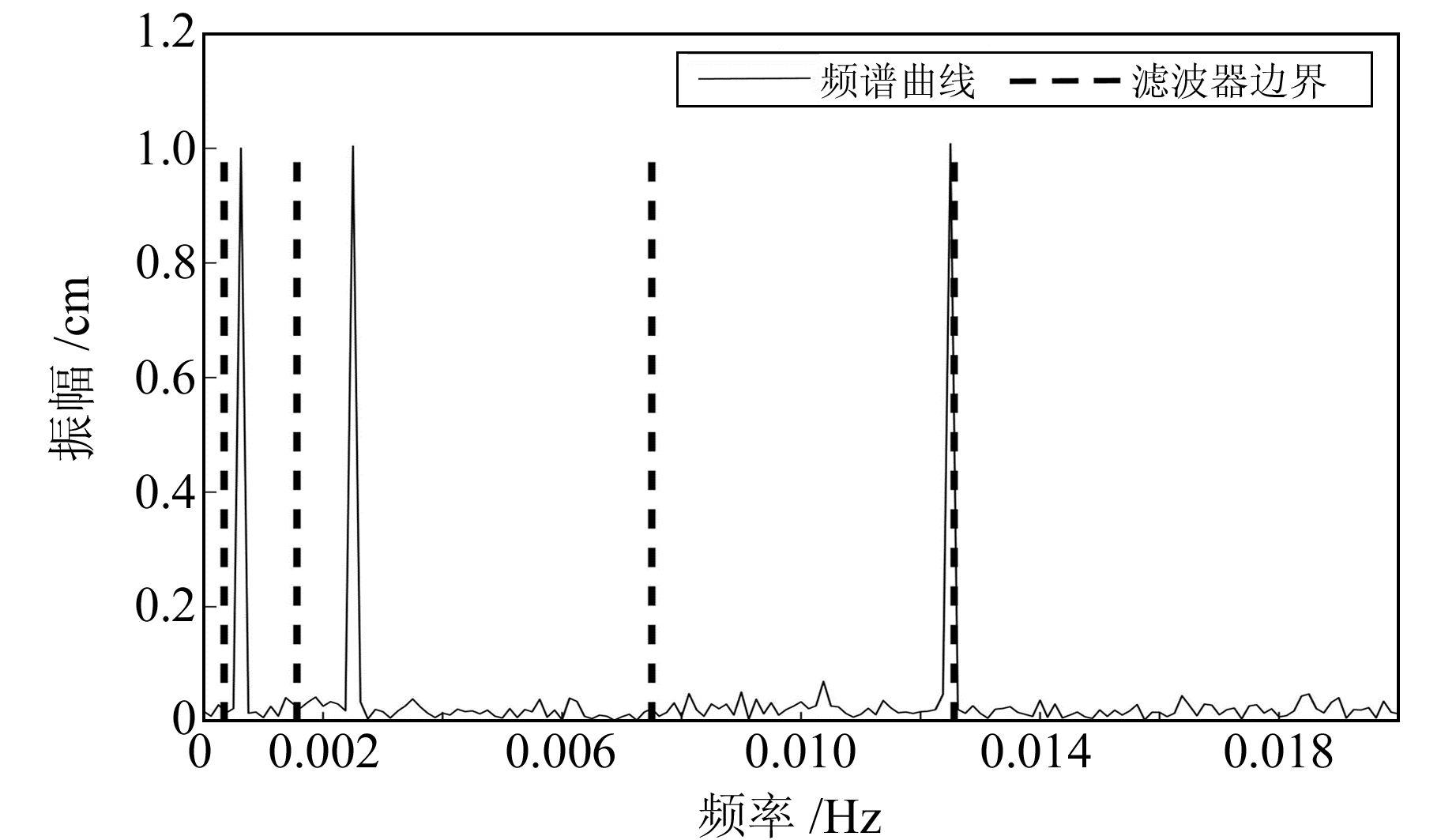图 1 仿真信号频谱图 Fig. 1 Spectrum of simulation signal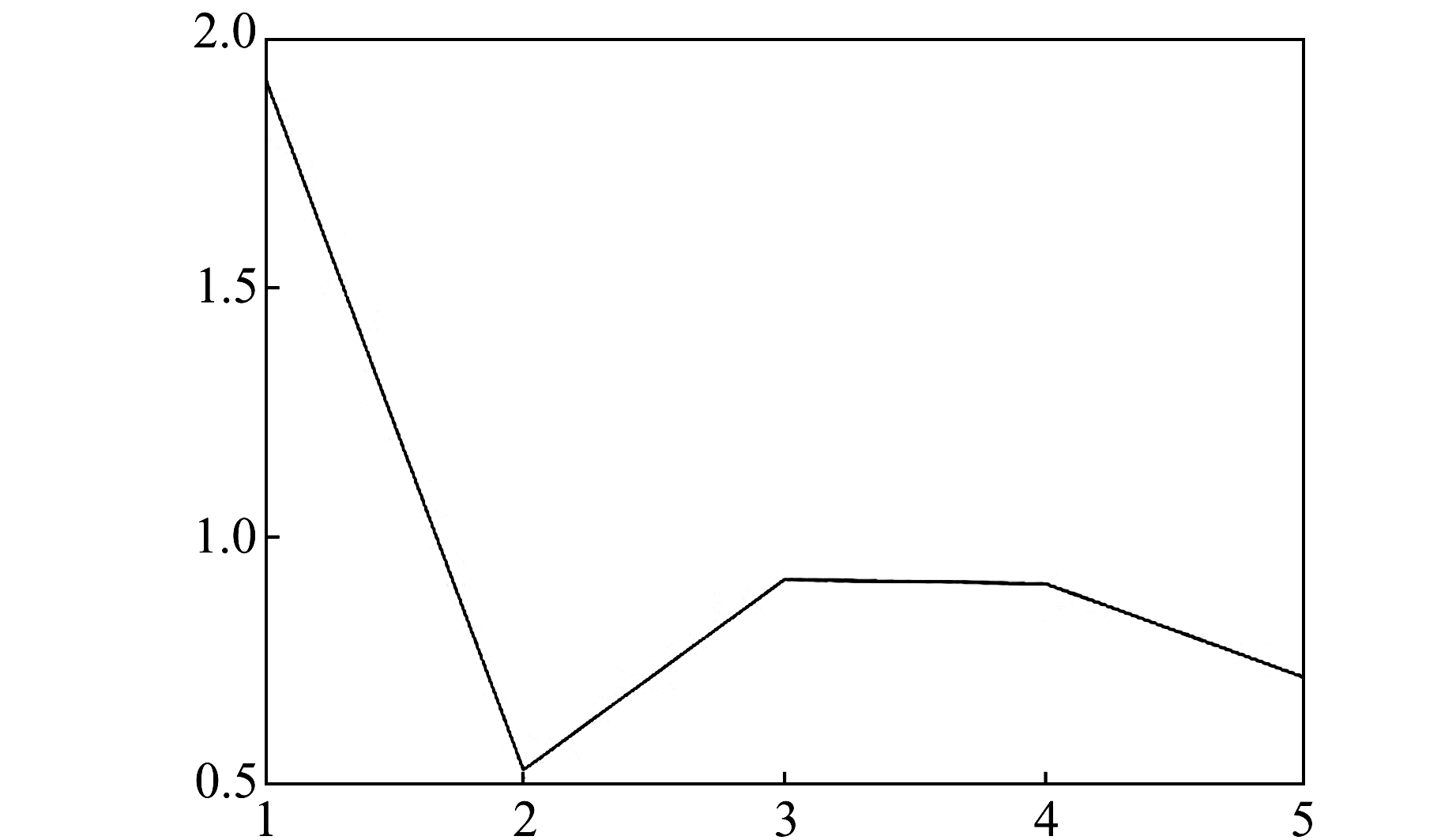图 2 仿真信号系数筛选 Fig. 2 Coefficient screening of simulation signal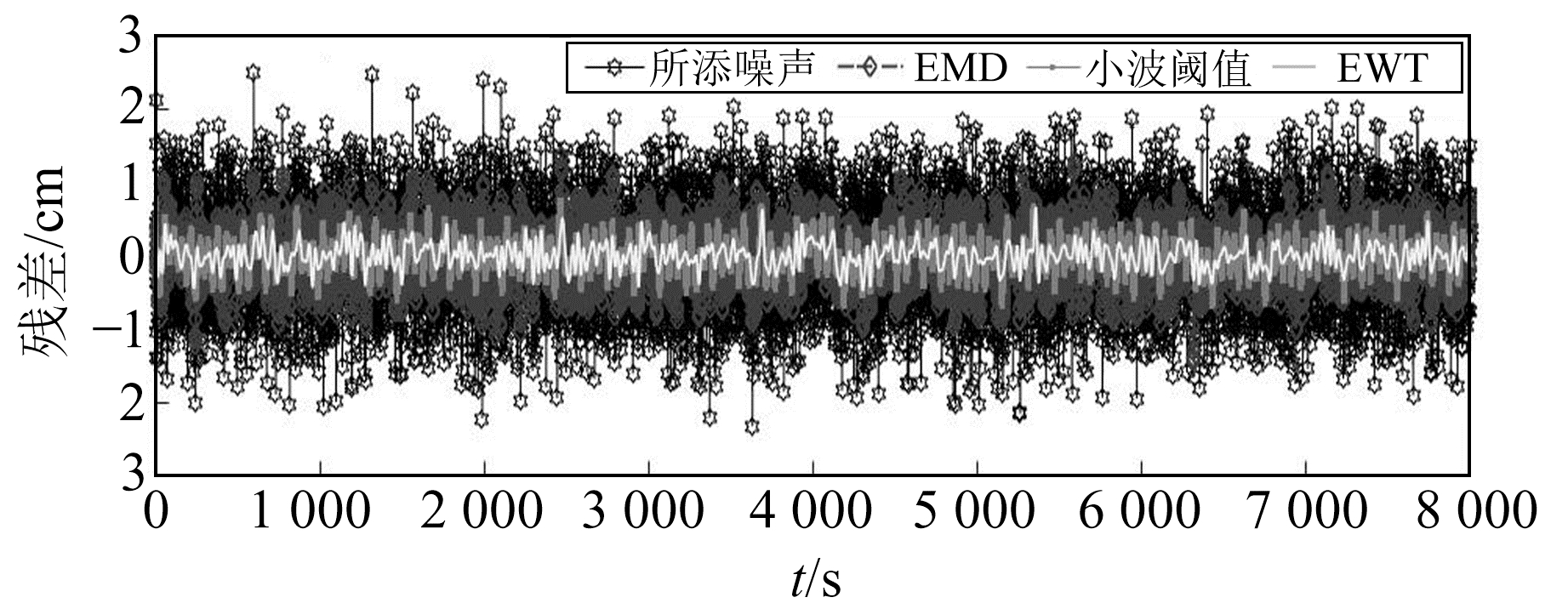图 3 去噪信号与原始参考信号的残差结果 Fig. 3 The residual results of denoised signal and original reference signal表 1 基于不同算法的降噪效果统计 Tab. 1 Statistics of denoising effectbased on different algorithms

2.2 GNSS实测数据结果与分析表 2 坐标残差序列相关系数 Tab. 2 Correlation coefficient of coordinate residual sequence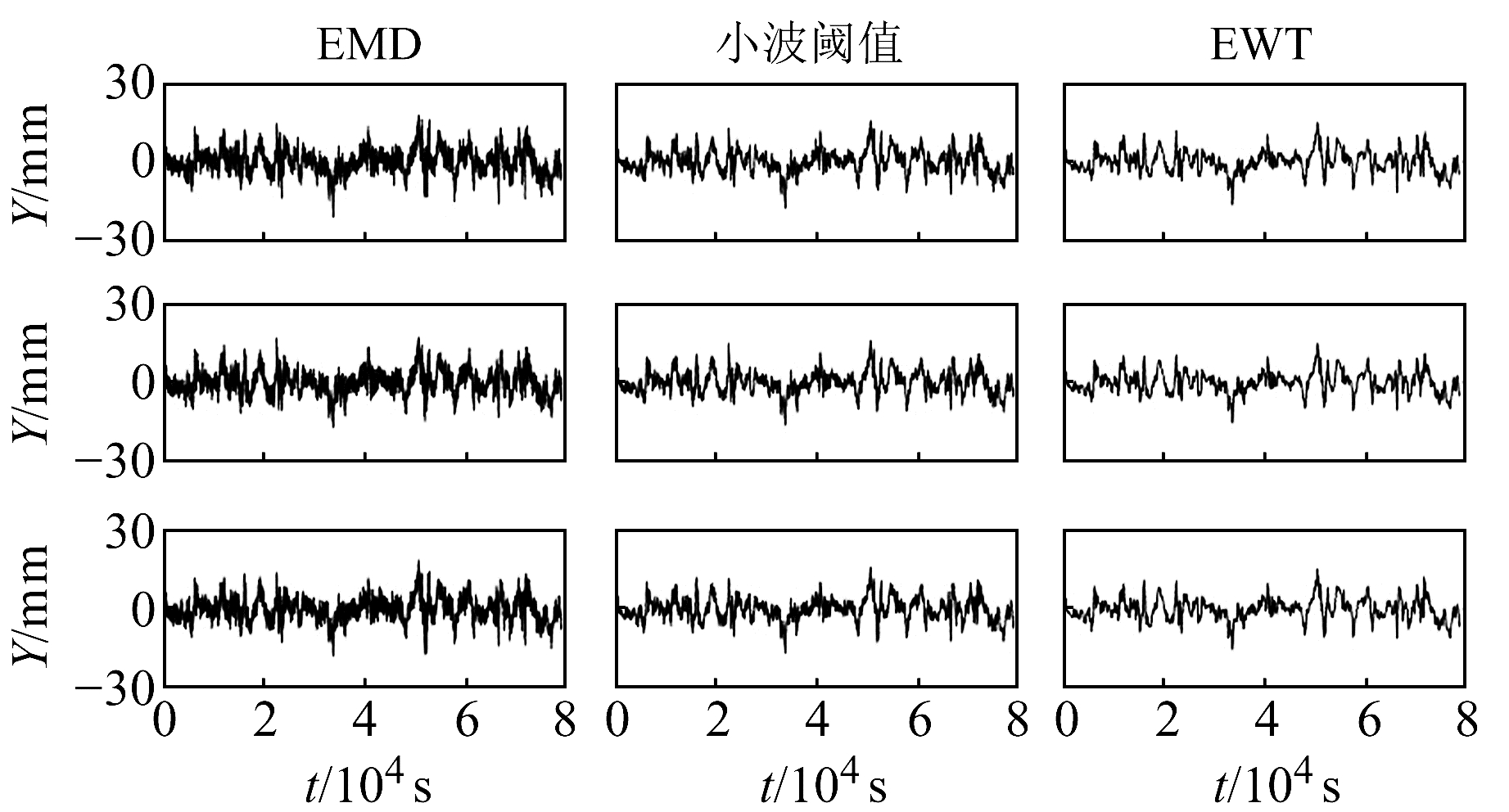图 4 3个算法提取的多路径模型序列 Fig. 4 Multipath model sequence extracted by three algorithms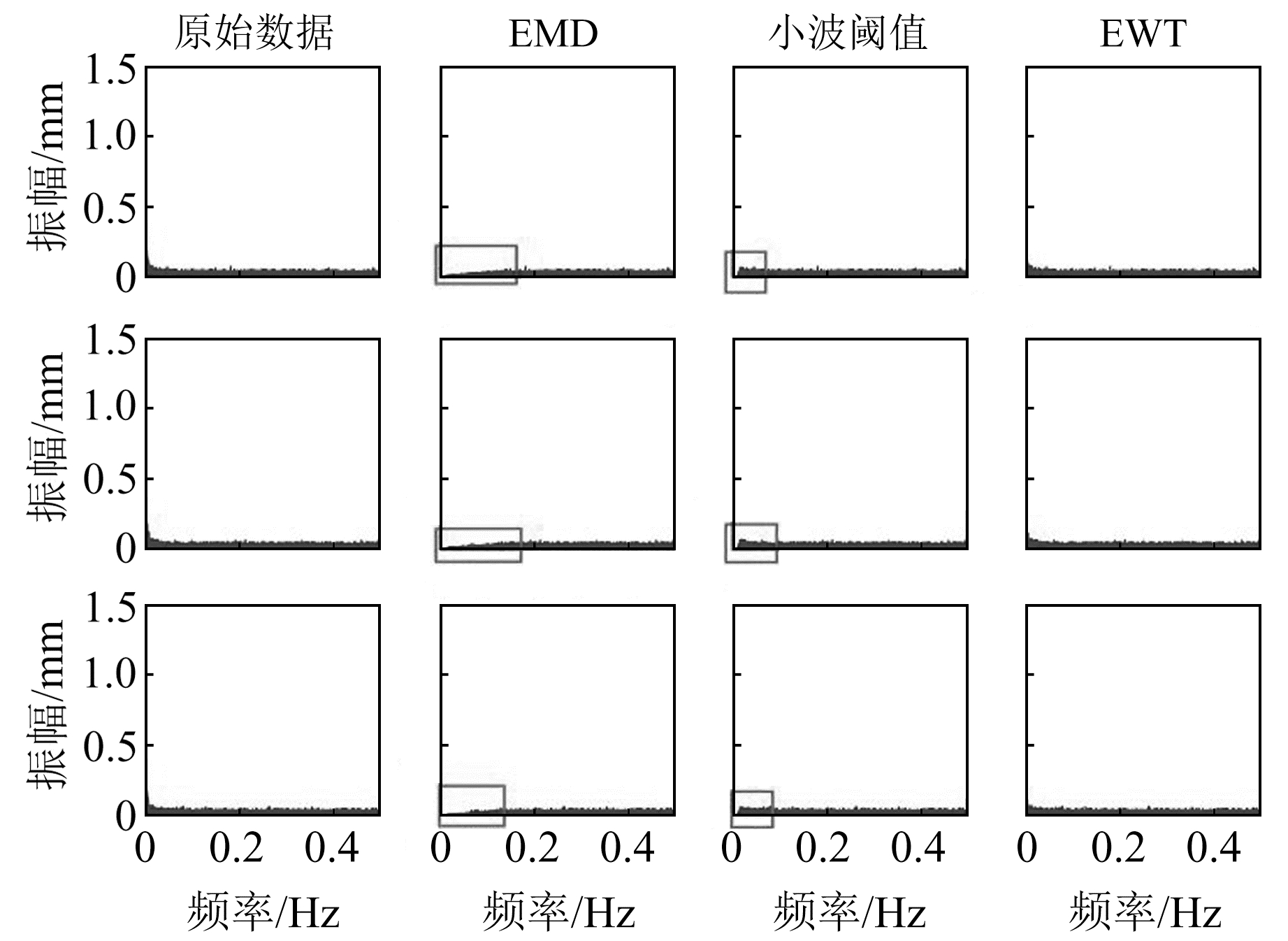图 5 3个算法去除的噪声频域图 Fig. 5 Noise frequency domain removed by three algorithms表 3 原始数据及提取的多路径误差模型的RMSE值 Tab. 3 RMSE values of original data and extracted multipath error model表 4 EMD、小波方法及EWT提取的3 d多路径误差模型的相关性 Tab. 4 Correlation coefficient of three days multipath error model extracted by EMD, wavelet method and EWT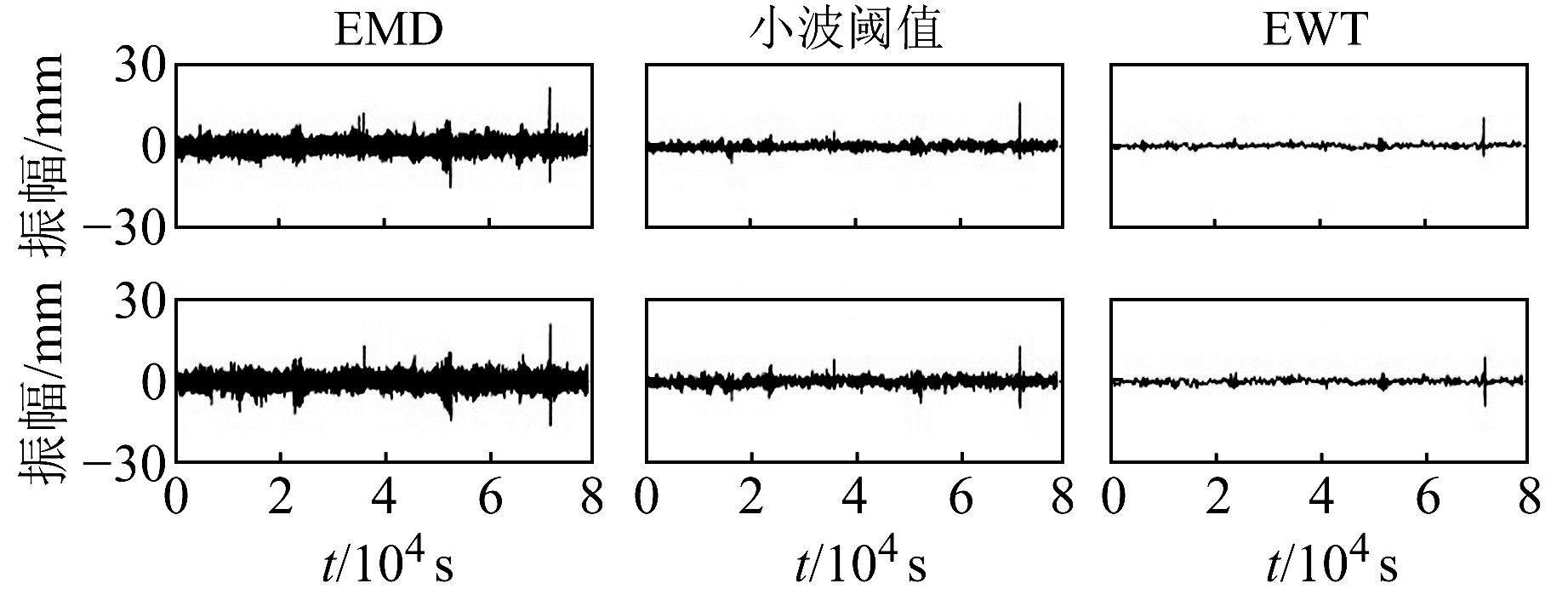图 6 Y方向3 d多路径误差残差序列 Fig. 6 Residual sequence of three days multipath error in Y direction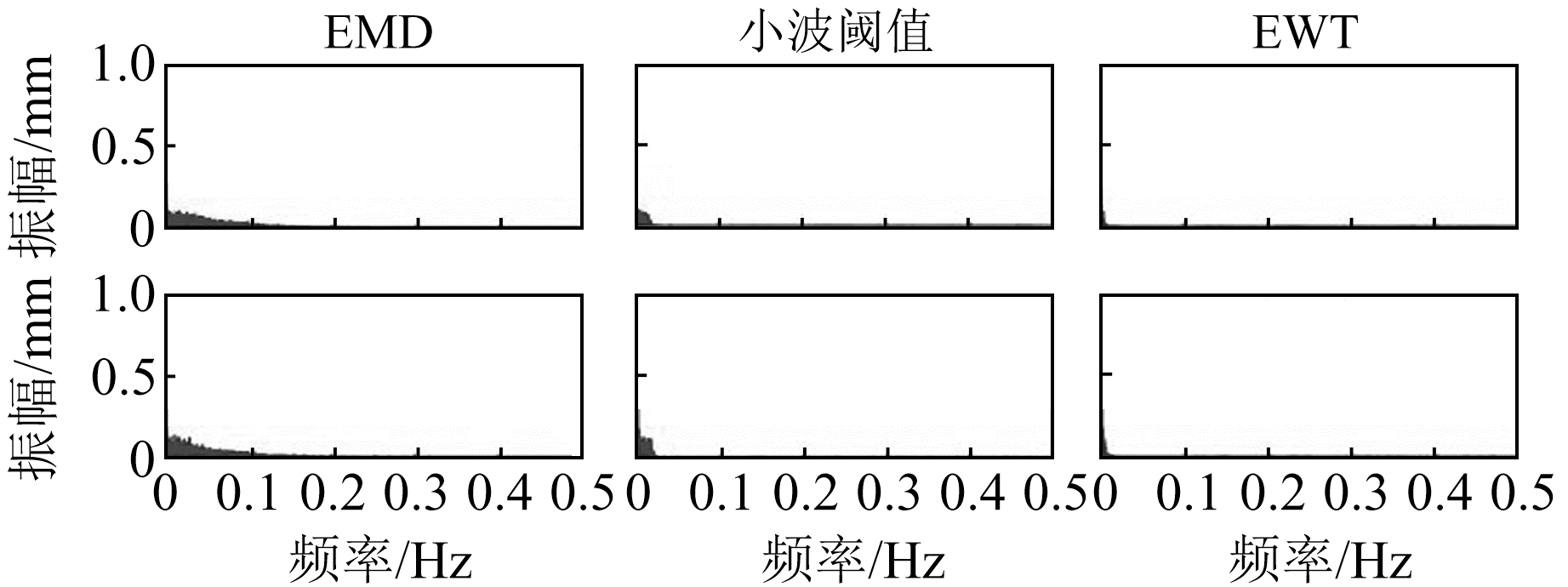图 7 多路径误差模型改正后的残差序列频谱图 Fig. 7 Residual sequence spectrum of multipath error model after correction表 5 多路径误差模型改正残差的RMSE值 Tab. 5 RMSE values of residual sequence corrected by multipath error model
3 结语

  严超, 王庆, 杨高朝, 等. EMD-RLS联合滤波算法及其在北斗多路径削弱误差中的应用[J]. 中国惯性技术学报, 2019, 27(2): 190-198 (Yan Chao, Wang Qing, Yang Gaochao, et al. EMD-RLS Combination Algorithm and Its Application in Weakening BDS Multipath Error[J]. Journal of Chinese Inertial Technology, 2019, 27(2): 190-198) (0)  Dong D N, Wang M H, Chen W, et al. Mitigation of Multipath Effect in GNSS Short Baseline Positioning by the Multipath Hemispherical Map[J]. Journal of Geodesy, 2016, 90(3): 255-262 (0)  Satirapod C, Rizos C. Multipath Mitigation by Wavelet Analysis for GPS Base Station Applications[J]. Survey Review, 2005, 38(295): 2-10 DOI:10.1179/sre.2005.38.295.2 (0)  Su M K, Zheng J S, Yang Y X, et al. A New Multipath Mitigation Method Based on Adaptive Thresholding Wavelet Denoising and Double Reference Shift Strategy[J]. GPS Solutions, 2018, 22(2): 40 DOI:10.1007/s10291-018-0708-z (0)  戴吾蛟, 丁晓利, 朱建军, 等. 基于经验模式分解的滤波去噪法及其在GPS多路径效应中的应用[J]. 测绘学报, 2006, 35(4): 321-327 (Dai Wujiao, Ding Xiaoli, Zhu Jianjun, et al. EMD Filter Method and Its Application in GPS Multipath[J]. Acta Geodaetica et Cartographica Sinica, 2006, 35(4): 321-327) (0)  Huang N E, Shen Z, Long S R, et al. The Empirical Mode Decomposition and the Hilbert Spectrum for Nonlinear and Non-Stationary Time Series Analysis[J]. Proceedings of the Royal Society A:Mathematical, Physical and Engineering Sciences, 1998, 454(1971): 903-995 DOI:10.1098/rspa.1998.0193 (0)  Li H L, Kwong S, Yang L H, et al. Hilbert-Huang Transform for Analysis of Heart Rate Variability in Cardiac Health[J]. IEEE/ACM Transactions on Computational Biology and Bioinformatics, 2011, 8(6): 1557-1567 DOI:10.1109/TCBB.2011.43 (0)  王琰, 张传定, 胡小工, 等. 一种地面连续运行参考站实时质量控制算法[J]. 仪器仪表学报, 2016, 37(11): 2506-2513 (Wang Yan, Zhang Chuanding, Hu Xiaogong, et al. Real-Time Quality Control Algorithm for Ground Continuously Operation Reference Stations[J]. Chinese Journal of Scientific Instrument, 2016, 37(11): 2506-2513) (0)  Gilles J. Empirical Wavelet Transform[J]. IEEE Transactions on Signal Processing, 2013, 61(16): 3999-4010 DOI:10.1109/TSP.2013.2265222 (0)  张超, 陈建军, 郭迅. 基于EEMD能量熵和支持向量机的齿轮故障诊断方法[J]. 中南大学学报:自然科学版, 2012, 43(3): 932-939 (Zhang Chao, Chen Jianjun, Guo Xun. Gear Fault Diagnosis Method Based on Ensemble Empirical Mode Decomposition Energy Entropy and Support Vector Machine[J]. Journal of Central South University:Science and Technology, 2012, 43(3): 932-939) (0)
GNSS Multipath Error Correction Based on Empirical Wavelet Transform
LUO Yiyong1,2CHEN Qiang1     HUANG Cheng1
1. Faculty of Geomatics, East China University of Technology, 418 Guanglan Road, Nanchang 330013, China;
2. School of Geodesy and Geomatics, Wuhan University, 129 Luoyu Road, Wuhan 430079, China
Abstract: Multipath error is the main factor affecting the measurement accuracy of GNSS and the difficulty of error correction. Combining the advantages of empirical wavelet transform(EWT) algorithm in the decomposition of complex signals with the daily repetition characteristics of GNSS multipath error, we propose a new method of multipath error extraction and correction based on EWT. The screening coefficient is defined by combining the energy entropy with the correlation coefficient, and the points with significant changes are taken as the dividing points of the noisy components and the information components. A new denoising method based on EWT is established by denoising the noisy components and reconstructing the information components. Through the analysis of simulation and experiment data, it is shown that EWT can extract and correct the GNSS multipath error better than EMD and wavelet method.
Key words: empirical wavelet transform; multipath error; denoising; GNSS